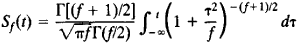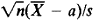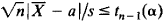# Student's Distribution

## Student's distribution

[′stüd·əns ‚dis·trə′byü·shən]
(statistics)
The probability distribution used to test the hypothesis that a random sample of n observations comes from a normal population with a given mean.

## Student’s Distribution

(or t distribution). Student’s distribution with f degrees of freedom is the distribution of the ratio t = X/Y of two independent random variables X and Y such that X is normally distributed with mathematical expectation EX = 0 and variance DX = 1 and fY2 has a chi-square distribution with f degrees of freedom.

The distribution function for t isLet X1, ..., Xn be independent random variables with the same normal distribution. If EXi, = a and DXi = σ2 (i = 1,..., n), then, for any real values of a and for σ > 0, the ratiohas a Student’s distribution with f = n – 1 degrees of freedom; here, = ∑Xi/n and s2 = ∑(Xi)/(n – 1). This property of the ratio was first made use of in 1908 by the British statistician W. Gosset to solve an important problem in classical error theory. The problem involves the testing of the hypothesis a = a0, where a0 is a given number and the variance σ2 is assumed to be unknown. If the inequalityis satisfied, the hypothesis a = a0 is regarded as not contradicting the observation results X1..., Xn. If the inequality does not hold, the hypothesis is rejected. Such a test involving the use of t is known as Student’s test, or the t test. The critical value t = tn–1 (α) is the solution of the equation Sn–1(t) = 1 - α/2, where α is a specified significance level (0 < α < ½). If the hypothesis a = a0 is true, then a is the probability that the hypothesis will be rejected when the test is performed with the critical value tn–1(α).

Student’s distribution is used in solving many other problems in mathematical statistics (seeSAMPLE, SMALL; ERRORS, THEORY OF; and LEAST SQUARES, METHOD OF).

### REFERENCE

Cramér, H. Matematicheskie metody statistiki, 2nd ed. Moscow, 1975. (Translated from English.)
Site: Follow: Share:
Open / Close